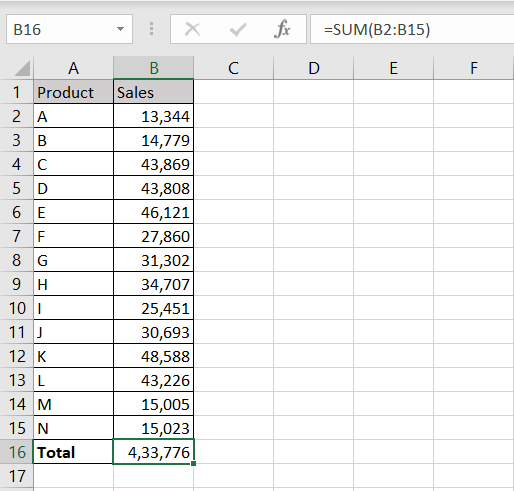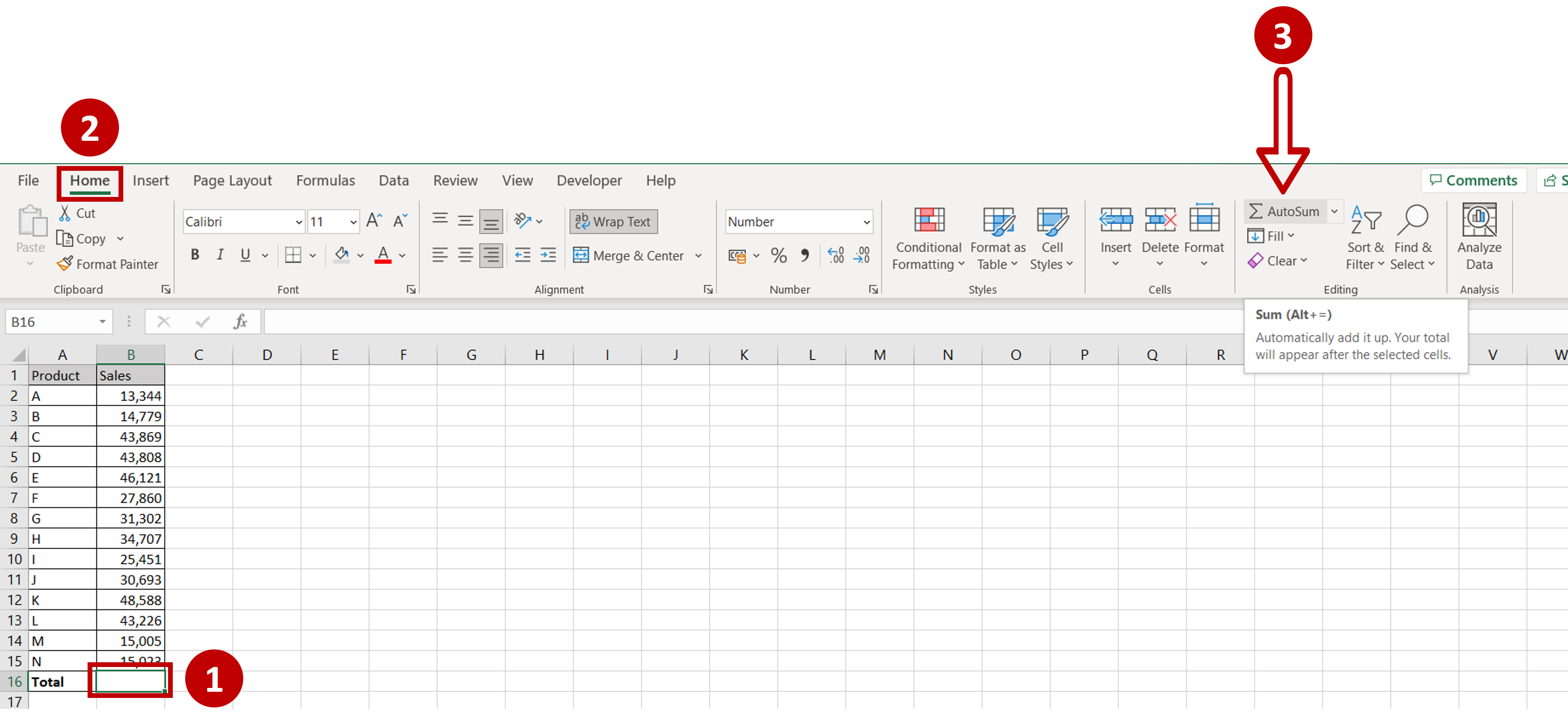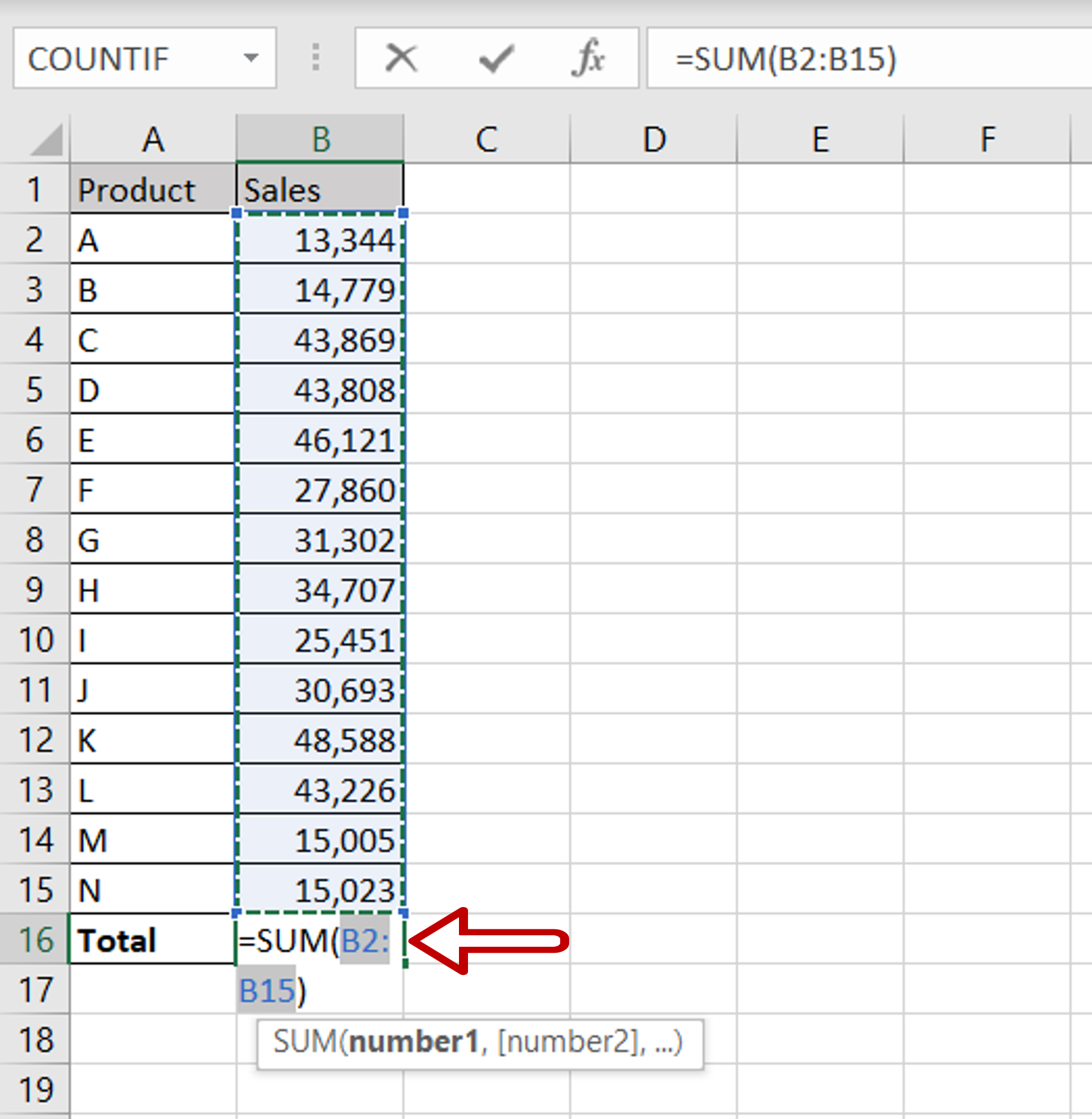# How to get the total of a column in Excel

You can watch a video tutorial here.

Excel is a great tool for performing calculations on tables. Using cell references in functions, you can create formulas for totals that will be updated whenever the source numbers change. This is simpler than using the addition operator (+) to add each number individually.Assume you have a table that has the sales for a set of products and you need to find the total sales for all products. The sales for each product are in a column, so you need to add all the numbers in the column. This can be easily done using AutoSum which uses the SUM()function.

### Step 1 – Click the AutoSum button– Select the first blank cell after the numbers in the column
– Go to Home > Editing
– Click the AutoSum button

### Step 2 – Check the formula– The SUM() function with the range of cells will be automatically populated in the cell
– Check that the range is correct
– Press Enter
Note: AutoSum will automatically populate the range only if the cell for the result is adjoining the range. If the cell for the result is elsewhere on the sheet, you will need to select the range of cells for the formula.

### Step 3 – Check the result– The total of the column is displayed